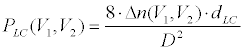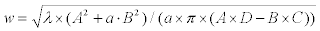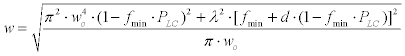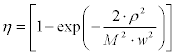An Electrically Tunable Liquid Crystal Lens for Fiber Coupling and Variable Optical Attenuation | OMICS International
Journal of Electrical & Electronic Systems

# An Electrically Tunable Liquid Crystal Lens for Fiber Coupling and Variable Optical Attenuation

Michael Chen, Chyong-Hua Chen, Yin-Chieh Lai, and Yi-Hsin Lin*

Department of Photonics, National Chiao Tung University, Hsinchu, 30010, R O C, Taiwan

*Corresponding Author:
Yi-Hsin Lin
Department of Photonics
National Chiao Tung University
Hsinchu, 30010, R O C, Taiwan
Tel: 886-3-5712121
E-mail: [email protected]

Received Date: February 07, 2014; Accepted Date: March 05, 2014; Published Date: March 07, 2014

Citation: Chen M, Chen CH, Lai YC, Lin YH (2014) An Electrically Tunable LiquidCrystal Lens for Fiber Coupling and Variable Optical Attenuation. J Electr Electron Syst 3:124. doi:10.4172/2332-0796.1000124

Copyright: © 2014 Chen M, et al. This is an open-access article distributed under the terms of the Creative Commons Attribution License, which permits unrestricted use, distribution, and reproduction in any medium, provided the original author and source are credited.

Visit for more related articles at Journal of Electrical & Electronic Systems

#### Abstract

An electrically tunable Liquid Crystal (LC) lens for both of fiber coupling and variable optical attenuation is demonstrated. The LC lens modulates the beam waist coupling to the fiber by electrically changing the lens power. When the modulated beam waist is close to the core size of the fiber, the LC lens is operated as a lens coupler. When the beam waist increases by reducing the lens power, the LC lens is operated as a Variable Optical Attenuator (VOA) as a result of the corresponding coupling coefficient variation of the transformed beam into a multimode fiber. The study provides a way to design an optical device for fiber coupling and variable optical attenuation based on electrically tunable focusing optical component.

#### Keywords

Liquid Crystal (LC) lens; lens coupler; Variable Optical Attenuator (VOA)

#### Introduction

Variable Optic Attenuators (VOAs) are indispensable components for optical power control in the applications of telecommunication systems, high speed data networks and optical sensor systems. Several technologies have been applied to implement VOAs based on the optical mechanisms of reflection, diffraction, interference, absorption, or scattering. Important examples include optical Micro- Electromechanical System (MEMS) technology , acousto-optics , Planar Lightwave Circuits (PLC) [3,4], and Liquid Crystal (LC) technology [5-8]. In fiber systems, VOA is usually used with another component, a lens coupler, which function is to collimate the light coming out of a fiber or to couple the light into a fiber. A conventional lens coupler usually consists of lenses with a fixed focal length. Tunable focusing properties of liquid lenses with an electrically adjusting curved membrane or interface can be used for fiber coupling or waveguide coupling [9,10]. However, Fresnel reflection from the interface between two fluids, the thickness of the liquid lens, the gravity issue, and the difficulty of encapsulation still limit their applications. Up to now, the literatures only discussed the function of the lens coupler by using a tunable focusing liquid lens in a fiber system [9,10].

To make fiber systems more compact, an electrically tunable device with combinative functions of VOA and lens coupler is very advantageous. With the superior property of modulating lights through electrically tunable molecular orientations, Liquid Crystal (LC) is a great candidate for electrically tunable devices. Several scattering-type VOAs have been proposed to reduce the input power by using polymerstabilized liquid crystals [6-8]. However, all these developed VOAs based on LC still require a lens coupler to couple the light into the fiber when applied to the fiber-connected optical systems. Our motivation is to design an electrically tunable LC device with functions of VOA and lens coupler for fiber-connected optical systems, which so far has not been realized. The LC phase modulator modulates the phase of the incident light. The lensing effect of the LC phase modulators is caused by the inhomogeneous spatial phase distribution originated from the inhomogeneous orientation distribution of the LC molecules, which can be controlled by the applied electric fields. The focal length of such a LC phase modulator or LC lens can be tunable as a positive lens or a negative lens depending on the electric fields [10,11]. LC lenses have various applications including image systems , pico-projector systems , optical zoom systems , concentrated photovoltaic systems , holographic systems , and ophthalmic lens .

In this paper, an electrically tunable LC lens for fiber coupling and variable optical attenuation is proposed and demonstrated. The LC lens electrically changes the lens power in order to adjust the beam size incident on the fiber. When the beam size is closed to the core size of the fiber, the LC lens is operated as a lens coupler. When the beam size increases by reducing the lens power, the LC lens is operated as a VOA. After introducing the operating principles, we use a LC lens to experimentally demonstrate the design concept and the results show that the maximum coupling efficiency can be 0.75 and the maximum attenuation can be as large as18 dB. The concept proposed in this paper is not only for LC lenses, but also for other optical components with electrically tunable focusing properties. The study provides a new way to design an optical device for fiber coupling and variable optical attenuation based on electrically tunable focusing optical components.

#### Mechanism and Operating Principles

Figure 1a and 1b depict a typical structure of the LC lens with positive and negative lens operations. We use the hole-patterned LC lens to demonstrate the concept [11,12]. In fact, the concept we proposed here can be applied to other structures of LC lenses. The components of the LC lens included three Indium Tin Oxide (ITO) glass substrates, two Polyvinyl Alcohol (PVA) layers for LC alignment, an insulating layer (Norland product Inc. NOA81), and a 50 μm nematic LC layer (Merck MLC-2070, Δn=0.2609 at 20°C at λ=589.3nm). In order to generate an inhomogeneous electric field, the ITO layer in the middle of the glass substrate was etched with a hole and connected to an applied Alternating Current (AC) voltage of V1. PVA layers were coated on the glass substrates and mechanically rubbed in one direction to align the LC molecules. As a result, the LC molecules are aligned parallel to the glass substrate (x-direction in Figure 1) as V1=V2=0Vrms and provide zero lens power (i.e. inverse of the focal length) for x-linearly polarized light. As V1>V2, the electric field near the peripheral region is stronger than in the center of the LC lens. As a result, the LC molecules at the center are more parallel to the x-direction and LC molecules near the edge are more parallel to the z-direction. Under this condition, the speed of x-linearly polarized light is slow in the center of the aperture and is fast in the peripheral region of the aperture. As a result, an incident plane wave of x-linearly polarized light is converted to a converged parabolic wave. The LC lens then provides a positive lens power with a parabolic spatial phase distribution, as illustrated in Figure 1a. Similarly, LC lens provides a negative lens power when V1<V2, as shown in Figure 1b. As long as the aperture size (D) and the thickness (dLC) of LC layer are fixed, the refractive indices difference (Δn) between the center and the peripheral region can decides the lens power of the LC lens (PLC), which can be written in the form of :(1)

Figure 1: The structure of the LC lens with (a) a positive lens mode and (b) a negative lens mode. (c) The x-linearly polarized incident beam converges when travels through the positive LC lens. The function of the LC lens is a lens coupler. (b) The x-linearly polarized incident beam diverges when travels through the negative LC lens. The function of the LC lens is VOA.

When V1>V2, the difference of refractive indices (Δn) is positive and the LC lens is operated as a positive lens. In addition, the lens power decreases as V2 increases while V1 is fixed. On the other hand, the LC lens is operated as a negative lens when V1<V2 and the lens power gradually increases while V1 increases and V2 is fixed.

Figure 1c and 1d illustrate the operating principle of a LC lens for both fiber coupling and variable optical attenuation. A Gaussian beam with a beam waist of wo impinges to a multimode fiber after propagating a distance of fmin (i.e. the minimum focal length). The distance between the beam waist (wo) and the LC lens is d and the lens power of the LC lens is denoted as PLC. Once we know the ABCD matrix of the system in Figure 1c and 1d, the beam waist (w) coupling to the fiber can be expressed as, where A,B,C and D are the elements in the ABCD matrix, λ is the optical wavelength, and a = λ ( π × wo2) . After we replace each element with the corresponding parameters mentioned above, the beam waist (w) can be written as a function of PLC:(2)

For a lens coupler whose Numerical Aperture (NA) is much smaller than NA of the fiber, the influence of incident angle can be neglected while calculating the coupling efficiency. In our experiments, NA of the fiber (~0.29) is much larger than NA of the LC lens (<0.02). Therefore, we assume that the coupling efficiency (η) is only determined by the ratio of the fiber core radius (ρ) to the beam waist (w), which is equal to the following :(3)

In Eq. (3), M2 is the beam propagation factor defined as π×w×θ/λ, where θ is the beam divergence of the incident wave. From Eq. (2) and Eq. (3), the coupling efficiency is determined by the incident beam waist (w), which can be continuously controlled by the electrically tunable lens power of the LC lens. As the lens power is relatively large (Figure 1c), the beam radius is similar to the fiber core radius, resulting in high coupling efficiency. The LC lens then works as a lens coupler. While the lens power decreases, the beam waist (w) is much larger than the radius of fiber core which reduces the optical power of the propagating light in the fiber. This means the optical attenuation can be adjusted by the LC lens. In Figure 1d, when a negative lens power is provided by the LC lens, the beam size is further enlarged and the optical attenuation range is also extended. Therefore, the LC lens can be a lens coupler and VOA at the same time. The mechanism illustrated above is applicable for either LC lenses with different structures or any tunable focusing devices whose lens power can be adjusted continuously from positive mode to negative mode.

#### Experiments and Discussions

In order to measure the lens power within an aperture of 1.33 mm under different applied voltages, the LC lens was placed between two crossed polarizers. The angles between the transmission axes of the polarizers and the rubbing direction were 45 degree. Therefore, the phase profiles with ring patterns were observed on the imaging plane as Figure 2a, 2b, and 2c. Figure 2a, and 2b show that the LC lens can either be a positive lens at (V1, V2)=(85Vrms, 0Vrms) or a negative lens at (V1, V2) =(0Vrms, 40Vrms). Figure 2c also displays a uniform phase profile at (V1, V2)=(85Vrms, 85Vrms) as the lens power of the LC lens was equal to zero. Two adjacent concentric rings in the phase profiles represent a phase difference of 2π radians. Based on Eq. (1), PLC can be rewritten as 8×N×λ/D2, where N is the number of concentric rings, λ is the operating wavelength, Δn×dLC equals N×λ, and D is the aperture size of the LC lens. Consequently, PLC under different applied voltages can be calculated from the corresponding phase profiles, as shown in Figure 2(d). The LC lens is a negative lens at V2=40Vrms>V1 while it works as a positive lens at V1=85Vrms>V2. For λ=633 nm, the evaluated lens power ranges from -8.9 m-1 to 30 m-1. As to the lens power in the infrared region, we use Cauchy’s equation, which indicates the dispersion relation of liquid crystal materials, to estimate the refractive indices of LC for the long optical wavelength . Typically, the refractive indices of extraordinary (ne) and ordinary (no) waves can be both expressed in the form of no,e=Ao,e+Bo,e-2+Co,e-4 with coefficients (Ae , Be, Ce) and (Ao , Bo, Co), respectively. The corresponding coefficients of MLC-2070 measured by Abbe refractometer are Ae=1.7180, Be=0.0119 μm2, Ce=0.0031μm4, Ao= 1.4899, Bo=0.0095 μm2, and Co =0.0002 μm4. Therefore, the lens power for λ=850 nm and λ=1300 nm can also be calculated from the results in visible region, which ranges are -8.3 m-1 ~28.5 m-1 and -8.1 m-1 ~27.7 m-1, respectively. As we can see in Figure 2d, the tunable lens power range decreases with the increasing optical wavelength because of the normal dispersion of MLC-2070.

Figure 2: (a) Coupling efficiency as a function of the lens power at different wavelengths. Red dots, blue squares and black triangles stand for λ=633nm, λ=850nm and λ=1300nm, respectively. (b) Attenuation as a function of the lens power at different wavelengths.(a)(b)(c) are the phase profiles of the LC lens observing under crossed-polarizers. λ=633nm. (a) The LC lens is a positive lens at (V1, V2) =(85Vrms, 0Vrms). f=1kHz (b) The LC lens is a negative lens at (V1, V2) =(0Vrms, 40Vrms). f=1kHz (c) Without lensing effect. P and A show the transmission axes of the polarizer and the analyzer, respectively. R indicates the rubbing direction. (d) The lens power as a function of applied voltages. Solid red dots, solid blue squares, and solid black triangles represent the positive lens power as a function of applied voltages of V2 at V1=85Vrms for three wavelengths of λ=633nm, λ=850nm, and λ=1300nm. Hollow red dots, hollow blue squares, and hollow black triangles represent the negative lens power as a function of applied voltages of V1 at V2=40Vrms for three wavelengths of λ=633nm, λ=850nm, and λ=1300nm.

In our experiments, we used three different laser sources for wavelengths of 633 nm, 850 nm, and 1300 nm: Melles Griot 05- srp-812, Power Technology APMT65 (850-100), and Agilent/HP 81552SM. Each laser passed through a single mode fiber (Thorlabs SM600 for visible light and Thorlabs SMF 28e+ for infrared light) and a lens collimator (Thorlabs F230FC-B for visible range, and Thorlabs F230FC-C for infrared range), which result in collimated incident beams with a diameter of 0.8 mm. In addition, a linear polarizer, whose transmission axis was parallel to the rubbing direction of the LC layer, was placed between each collimated laser beam and the LC lens. The incident light then coupled to a 30 cm long multimode fiber (Newport, F-MLD-500, core/cladding: 100 μm/140 μm diameter, NA=0.29) after passed through the LC lens. The distances between the LC lens and the fiber were decided by the minimum focal lengths at each wavelength, which were 3 cm as λ=633 nm, 3.3 cm as λ=850 nm and 3.4 cm as λ=1300 nm. The optical powers before and after transmitting through the fiber were detected by a power meter (OPHIR AN/2 for λ=633 nm and Newport 1916-R for λ=850 nm and λ=1300 nm). Therefore, we obtained the coupling efficiency (η) as a function of the lens power from the ratio of the measured powers, as shown in Figure 3a. Without the lens power of the LC lens, the coupling efficiencies are 0.027 for λ=633 nm, 0.025 for λ=850 nm and 0.031 for λ=1300 nm. When the lens power of the LC lens increases positively, the coupling efficiency also increases. The maximum coupling efficiencies are 0.75, 0.68, and 0.5 for wavelengths of 633 nm, 850 nm, and 1300 nm, respectively. This indicates the LC lens is operated as a lens coupler because more lights are collected into the fiber. When the lens power of the LC lens increases negatively, the coupling efficiency decreases because of the divergence of the beam coupled to the fiber. The coupling efficiencies are different for the same lens power at different wavelengths, simply because the coupling efficiency depends on not only the lens power but also the wavelength. We further plotted the optical attenuation as a function of the lens power, as shown in Figure 3b. The optical attenuation is defined as 10×log10 (η). In Figure 3b, the optical attenuation increases with a decrease of the lens power of the LC lens. This is because the beam size coupled into the fiber is larger as the lens power is smaller and then the optical attenuation is enhanced. The maximum attenuations are 17.3 dB at 633nm, 17.7 dB at 850 nm, and 16.7 dB at 1300 nm. Figure 3a and Figure 3b show that the LC lens can not only be an electrically tunable lens coupler but also an electrically tunable VOA.

Figure 3: (a) Coupling efficiency as a function of the lens power at different wavelengths. Red dots, blue squares and black triangles stand for λ=633nm, λ=850nm and λ=1300nm, respectively. (b) Attenuation as a function of the lens power at different wavelengths.

Under the assumption of an ideal Gaussian beam (M2=1), we can evaluate the beam waist (w) at different lens power from the known fiber core radius ρ=50 μm and Eq. (3). The results are plotted in Figure 4. Without focusing effect of the LC lens, the incident beams simply pass through and maintain their beam waists (~400 μm). Because the beam waists coupling the fiber are much larger than the core radius (50 μm), the coupling efficiency is low (~0.027) and the optical attenuation is high (~15.7dB), as shown in Figure 3a and 3b. When the lens power increases, the beam waist turns out smaller which results in higher coupling efficiency and lower optical attenuation. The smallest beam waists for wavelengths of 633 nm, 850 nm and 1300 nm are 60 μm, 68 μm and 85 μm, respectively. At this moment, the beam waists are similar to the core radius of the fiber which causes maximum coupling efficiency as well as minimum optical attenuation. Therefore, an electrically tunable liquid crystal lens can be operated as an electrically tunable fiber coupling device with both functions of lens coupler and VOA by manipulating the beam waist coupling to the fiber. We also plotted the theoretical values of beam waists in Figure 4 based on an assumption of perfect thin lens and the relative experimental parameters in Eq (2): w0=0.4 mm, fmin=33.0 mm for λ=633 nm, fmin=35.1 mm for λ=850 nm, fmin=36.1 mm for λ=1300 nm, d=12.5 cm for λ=633 nm, d=11.0 cm for λ=850 nm, and d=8.7 cm for λ=1300 nm. The theoretical minimum beam waists for 633 mm, 850 nm and 1300 nm are 16 μm, 24 μm and 37 μm, respectively. As a result, the theoretical minimum beam waists are smaller than the experimental values for all three wavelengths. This indicates that the phase profiles of the LC lens with large lens power are not identical to the parabolic distribution which is used in our theoretical model. Furthermore, scattering effect originated from inhomogeneous LC layer may also enlarge the beam waist. This explains the reason why the experimental beam waists cannot be smaller than the fiber core radius at the maximum lens power and restricts the maximum coupling efficiency to be lower than 0.8. In order to increase the coupling efficiency as well as optical attenuation, we not only need to optimize the LC lens structure for parabolic phase distribution, but also have to enlarge the tunable range in negative lens operation by using high bi-refringent LC materials or increasing the thickness of the LC layer. Moreover, the insertion loss of the lens was ~0.7dB due to multiple interface reflection and light scattering. This might be reduced by coating the anti-reflection layers on the LC lens.

Figure 4: Beam waist (w) as a function of lens power. Solid red dots, solid blue squares, and solid black triangles represent experimental results at different wavelengths of 633 nm, 850 nm, and 1300 nm. Red solid line, blue dotted line, and black dotted line represent the theoretical results at different wavelengths of 633 nm, 850 nm, and 1300 nm.

We also measured the response times of the LC lens. As the lens power switched from 0 m-1 to 30 m-1, the response time was 762 ms. On the other hand, the switching time was 382ms when the lens power was turned back to 0 m-1. In order to further improve the response time, we can either adopt the overdriving scheme of applied voltages or use polymer network LC materials . In addition, polymer-stabilized blue phase liquid crystals have been used to realize a LC lens with submilliseconds response time, which shows great potential for this study . The LC lens in this paper still requires high applied voltages. This can be solved by using many other structures of LC lens operated with a driving voltage below 10 Vrms [10,21]. Moreover, the optical efficiency can be enhanced by using polarization independent LC phase modulator, including double layered type, residual phase type or mixed type [17,20,22-30].

Compared to prior arts, the attenuation in the prior arts is around 30dB and polarization dependent loss less than 4dB with the response time of sub-ms~80 ms [1-7]. The VOA function we demonstrated is polarization dependent and the maximum attenuation is 15.7 dB with a switching time of ~1 sec. However, LC lens can provides the function of not only variable optical attenuation but also fiber coupling which differs from prior VOAs. Compared to typical lens couplers, such as GRIN lens coupler with a fixed focal length (low coupling loss ~0.1 dB), the LC lens not only serves as a lens coupler but also is able to control the attenuation level of optical power as a VOA due to an electrically tunable focal length of the LC lens. Therefore, the device we proposed outperforms other types of VOA because of its dual functionality in a single device.

#### Conclusion

A mechanism for fiber coupling and variable optical attenuation via an electrically tunable LC lens is demonstrated. The beam waist coupling to the fiber can be controlled by adjusting the lens power of the LC lens. Therefore, the coupling coefficient and optical attenuation can be changed due to the tunable lens power. We have shown the experimental results for three wavelengths from visible to near infrared range. For broad band fiber systems, compact LC lens is a good candidate as lens coupler and VOA. Since polarization dependency of the LC lens, polarization-maintaining optical fibers are required in the practical applications. This concept is also applicable for other optical devices with tunable focusing ability. This study has several potential applications, such as gain-flattened amplifying systems and optical power equalization systems.

#### Acknowledgements

This research was supported by the National Science Council (NSC) in Taiwan under the contract no. 101-2112-M-009-011-MY3.

#### References

Select your language of interest to view the total content in your interested language

### Article Usage

• Total views: 12859
• [From(publication date):
April-2014 - Jan 23, 2020]
• Breakdown by view type
• HTML page views : 9018Can't read the image? click here to refresh